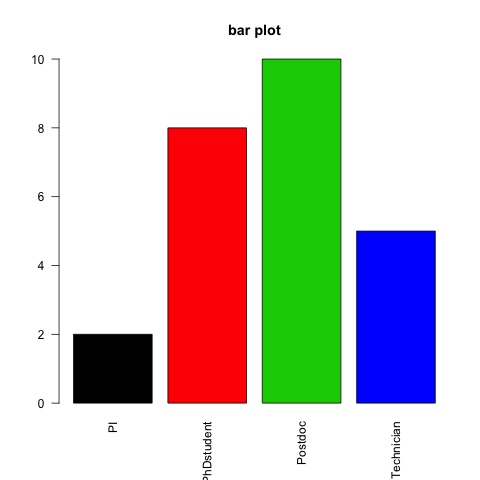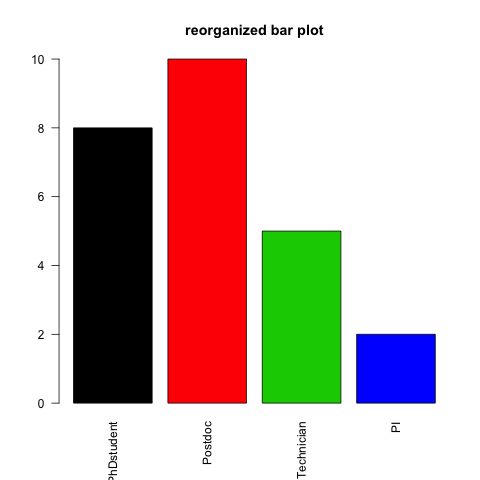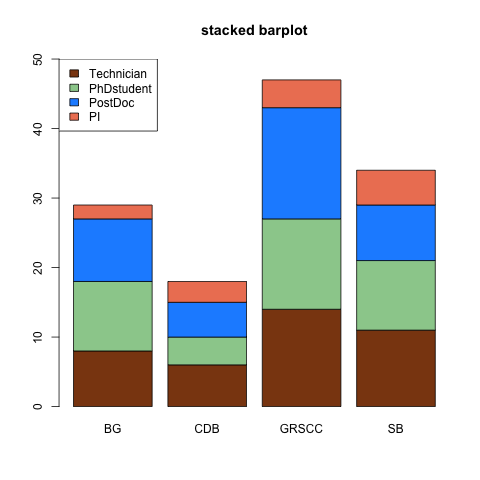## 16.2 Bar plots

A bar chart or bar plot displays rectangular bars with lengths proportional to the values that they represent.

• A simple bar plot :
``````# Create a vector
x <- rep(c("PhDstudent", "Postdoc", "Technician", "PI"), c(8,10,5,2))

# Count number of occurences of each character string
mytable <- table(x)

# Bar plot using that table
barplot(mytable)``````• Customize a bit :
• col : color
• main : title of the plot
• las : orientation of x-axis labels: “2”: perpendicular to axis
``````barplot(mytable,
col=1:4,
main="bar plot",
las=2)``````• Customize the ordering of the bars :

By default, the bars are organized in alphabetical order. You can change it with the factors.

``````# Create an ordered factor out of x
xfact <- factor(x,
levels=c("PhDstudent", "Postdoc", "Technician", "PI"),
ordered=TRUE)

# Produce the table
xfacttable <- table(xfact)

# Plot the same way
barplot(xfacttable,
col=1:4,
main="reorganized bar plot",
las=2)``````• Let’s make a stacked barplot :
``````# Create a matrix of number of type of employees per research program :
barmat <- matrix(c(8, 10, 9, 2, 6, 4, 5, 3, 14, 13, 16, 4, 11, 10, 8, 5),
nrow=4,
dimnames=list(c("Technician", "PhDstudent", "PostDoc", "PI"), c("BG", "CDB", "GRSCC", "SB")))

# Plot barplot
barplot(barmat, col=sample(colors(), 4))``````
``````# set a random color vector
mycolors <- sample(colors(), 4)

# plot barplot
# ylim sets the lower and upper limit of the y-axis: here it allows us to fit the legend !
barplot(barmat,
col=mycolors,
ylim=c(0,50),
main="stacked barplot")